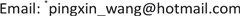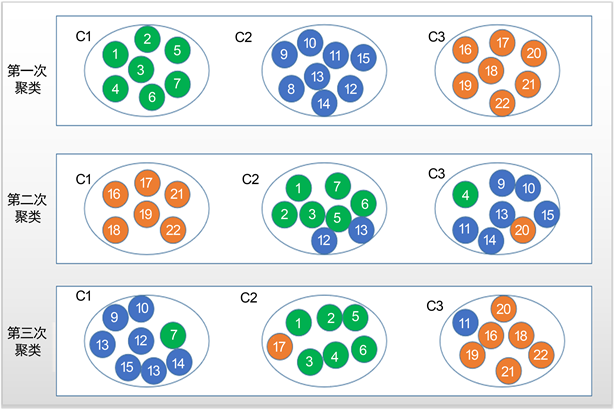﻿ 基于投票理论的三支聚类分析 Three-Way Clustering Analysis Based on Voting Theory

Computer Science and Application
Vol. 09  No. 12 ( 2019 ), Article ID: 33548 , 8 pages
10.12677/CSA.2019.912261

Three-Way Clustering Analysis Based on Voting Theory

Liuquan Gu, Ruilin Chai, Pingxin Wang*

School of Science, Jiangsu University of Science and Technology, Zhenjiang JiangsuReceived: Dec. 2nd, 2019; accepted: Dec. 13th, 2019; published: Dec. 20th, 2019ABSTRACT

At present, most of existed clustering methods are two-way clustering which are based on the assumption that a cluster must be represented by a set with crisp boundary. However, assigning uncertain objects into a certain cluster will reduce the accuracy of clustering results. Three-way clustering is an overlapping clustering which describes each cluster by core region and fringe region. It handles the category problem of uncertain objects and reduces the decision risk effectively. This paper mainly introduces a model of three-way clustering, and gives three-way clustering algorithm based on k-means as an example for analysis. Firstly, different clustering results of the same data set are obtained by ensemble clustering. Then, the label matching method is used. Finally, the cluster of objects is determined according to the voting rules. Through the analysis of experimental results, it is verified that the effect of the clustering method has been significantly improved.

Keywords:K-Means, Three-Way Clustering, Label Matching, Voting Rules1. 引言

2. 相关工作

2.1. 三支聚类

$C\left({m}_{i}\right)\cup F\left({m}_{i}\right)\cup T\left({m}_{i}\right)=U\text{\hspace{0.17em}}\text{\hspace{0.17em}}\left(i=1,2,\cdots ,k\right)$ (1)

$\underset{i=1}{\overset{k}{\cup }}\left(C\left({m}_{i}\right)\cup F\left({m}_{i}\right)\right)=U\text{\hspace{0.17em}}\text{\hspace{0.17em}}\left(i=1,2,\cdots ,k\right)$ (2)

$C\left({m}_{i}\right)\ne \varphi \text{\hspace{0.17em}}\text{\hspace{0.17em}}\left(i=1,2,\cdots ,k\right)$ (3)

2.2. 匹配原则Figure 1. Schematic diagram of clustering results

2.3. 投票原则

$f\left({x}_{i}\right)=\frac{{m}_{j}}{N}$ (4)

$g\left({x}_{i}\right)=\left\{\begin{array}{ll}{x}_{i}\in C\left({m}_{j}\right),\hfill & \text{\hspace{0.17em}}\text{\hspace{0.17em}}\text{\hspace{0.17em}}f\left({x}_{i}\right)\ge 0.9\hfill \\ {x}_{i}\in F\left({m}_{j}\right),\hfill & 0 (5)

3. 聚类结果评价指标

3.1. 准确率

$\text{ACC}=\frac{1}{N}\underset{i=1}{\overset{k}{\sum }}{M}_{i}$ (6)

3.2. Davies-Bouldin Index

Davies-Bouldin index通过计算每个类簇最大相似度的均值，是一种评估聚类算法优劣的内部聚类评价指标。使用下列公式进行计算

$\text{DBI}=\frac{1}{N}\underset{i=1}{\overset{N}{\sum }}\underset{i\ne j}{\mathrm{max}}\left(\frac{\stackrel{¯}{{S}_{i}}+\stackrel{¯}{{S}_{j}}}{{‖{w}_{i}-{w}_{j}‖}_{2}}\right)$ (7)

$\stackrel{¯}{{S}_{i}}={\left(\frac{1}{{T}_{i}}\underset{j=1}{\overset{{T}_{i}}{\sum }}{|{x}_{j}-{A}_{j}|}^{p}\right)}^{\frac{1}{p}}$ (8)

${x}_{j}$ 代表类簇i中第j个样本点， ${A}_{i}$ 是类簇i的质心， ${T}_{i}$ 是类簇i中样本的个数，p在通常情况下取值为2。

4. 实验数据分析

5. 结语

Three-Way Clustering Analysis Based on Voting Theory[J]. 计算机科学与应用, 2019, 09(12): 2349-2356. https://doi.org/10.12677/CSA.2019.912261

1. 1. Li, J.H., Huang, C.C., Qi, J.J., Qia, Y.H. and Liu, W.Q. (2017) Three-Way Cognitive Concept Learning via Mul-ti-Granularity. Information Sciences, 378, 244-263. https://doi.org/10.1016/j.ins.2016.04.051

2. 2. Lingras, P. and Yan, R. (2004) Interval Clustering Using Fuzzy and Rough Set Theory. IEEE Annual Meeting of the Fuzzy Information, Banff, 27-30 June 2004, 780-784. https://doi.org/10.1109/NAFIPS.2004.1337401

3. 3. Liu, D., Yao, Y.Y. and Li, T.R. (2011) Three-Way Investment Decisions with Decision-Theoretic Rough Sets. International Journal of Computa-tional Intelligence Systems, 4, 66-74. https://doi.org/10.1080/18756891.2011.9727764

4. 4. Li, Y., Zhang, C. and Swanb, J.R. (2000) An Information Filtering on the Web and Its Application in Job Agent. Knowledge-Based Systems, 13, 285-296. https://doi.org/10.1016/S0950-7051(00)00088-5

5. 5. Yao, Y.Y. (2010) Three-Way Decisions with Probabilistic Rough Sets. Information Sciences, 180, 341-353. https://doi.org/10.1016/j.ins.2009.09.021

6. 6. Yao, Y.Y. (2011) The Superiority of Three-Way Decisions in Probabilistic Rough Set Models. Information Sciences, 181, 1080-1096. https://doi.org/10.1016/j.ins.2010.11.019

7. 7. Yao, Y.Y. (2012) An Outline of a Theory of Three-Way Decisions. In: Yao, J., Yang, Y., et al., Eds., Rough Sets and Current Trends in Computing, Springer, Berlin, Heidelberg, Vol. 7413, 1-17. https://doi.org/10.1007/978-3-642-32115-3_1

8. 8. Yu, H., Chu, S.S. and Yang, D.C. (2010) Autonomous Knowledge-Oriented Clustering Using Decision-Theoretic Rough Set Theory. Rough Set and Knowledge Technology, In: Yu, J., Greco, S., Lingras, P., Wang, G. and Skowron, A., Eds., Springer, Berlin, Heidelberg, Vol. 6401, 687-694. https://doi.org/10.1007/978-3-642-16248-0_93

9. 9. Yu, H., Liu, Z.G. and Wang, G.Y. (2014) An Automatic Method to Determine the Number of Clusters Using Decision-Theoretic Rough Set. International Journal of Approxi-mate Reasoning, 55, 101-115. https://doi.org/10.1016/j.ijar.2013.03.018

10. 10. Yu, H., Zhang, C. and Wang, G.Y. (2016) A Tree-Based Incremen-tal Overlapping Clustering Method Using the Three-Way Decision Theory. Knowledge Based Systems, 91, 189-203. https://doi.org/10.1016/j.knosys.2015.05.028

11. 11. Yu, H., Jiao, P., Yao, Y.Y., et al. (2016) Detecting and Refining Overlapping Regions in Complex Networks with Three-Way Decisions. Information Sciences, 373, 21-41. https://doi.org/10.1016/j.ins.2016.08.087

12. 12. 于洪. 三支聚类分析[J]. 数码设计, 2016, 5(1): 31-35.

13. 13. Macqueen, J. (1967) Some Methods for Classification and Analysis of Multivariate Observations. Proceed-ings of the Fifth Berkeley Symposium on Mathematical Statistics and Probability, Berkeley, 1967, 281-297.

14. 14. Schölkopf, B., Platt, J. and Hofmann, T. (2006) A Local Learning Approach for Clustering. International Conference on Neural Information Processing Systems, MIT Press, 2007, 1529-1536.

15. 15. Davies, D.L. and Bouldin, D.W. (1979) A Cluster Separation Measure. IEEE Transactions on Pattern Analysis & Machine Intelligence, 1, 224-227. https://doi.org/10.1109/TPAMI.1979.4766909

16. NOTES

*通讯作者。